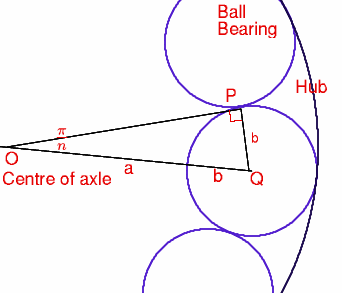You may also likeOverarch 2

Bricks are 20cm long and 10cm high. How high could an arch be built without mortar on a flat horizontal surface, to overhang by 1 metre? How big an overhang is it possible to make like this?Cushion Ball

The shortest path between any two points on a snooker table is the straight line between them but what if the ball must bounce off one wall, or 2 walls, or 3 walls?Stonehenge

Explain why, when moving heavy objects on rollers, the object moves twice as fast as the rollers. Try a similar experiment yourself.

Ball Bearings

Age 16 to 18 Challenge Level:

Sue Liu of Madras College, St Andrews sent an excellent solution to this question.

Suppose that $a$ is the radius of the axle, $b$ is the radius of each ball-bearing, and $c$ is the radius of the hub (see the figure).

The solution is based on the following figure.The angle subtended by each ball-bearing at the centre of the axle is $2\pi/n$, so that in the triangle $OPQ$ we have $$OQ= a+b, \quad PQ = b, \quad \angle POQ = \pi/n$$ and hence
1. $$(a+b)\sin (\pi/n) = b.$$
We also know that
1. $$c = OP' = OP +PP' = a+2b.$$
The rest of the solution is applying simple algebra to (1) and (2), and to simplify this we will (temporarily) write $s$ for $\sin (\pi/n)$. First, (1) gives $$as = b(1-s).$$ Next, (2) gives $$c = a+2b = a + {2as\over 1-s} = a\left({1+s\over 1-s}\right),$$ so that, finally, we have $${a \over b} = \left({1 - \sin (\pi/n)\over \sin (\pi /n)}\right), \quad {b \over c} = \left({\sin (\pi/n)\over 1+\sin (\pi /n)}\right), \quad {c\over a} = \left({1+\sin (\pi/n)\over 1-\sin (\pi /n)}\right).$$ When there are 3 ballbearings, $n = 3$ and $\sin (\pi /3) = \sqrt 3 /2$. Hence$${a \over b}= {2\sqrt 3 \over 3} - 1, \quad {b \over c} = \sqrt 3(2 - \sqrt 3), \quad {c \over a} = 7 + 4\sqrt 3.$$ When there are 4 ballbearings, $n = 4$ and $\sin (\pi /4) = \sqrt 1 /\sqrt 2$. Hence $${a \over b}= \sqrt 2 - 1, \quad {b \over c} = \sqrt 2 - 1, \quad {c \over a} = 3 + 2\sqrt 2.$$ {\bf If $n=6$ then} $b = a$ and $c = 3a$. This is a very special case for suppose, in the general case, that the internal radius $c$ of the hub is an integer multiple of the radius $b$ of each ball-bearing. Then $c/b = N$, say, where $N$ is an integer, and this gives $$N\sin {\pi \over n} = 1+\sin {\pi\over n},$$ or $$\sin {\pi\over n} = {1\over N-1}.$$ Now it is known (although this is NOT an elementary result) that if $\sin x$ is rational, then $\sin x$ is $0$, $1/2$ or $1$. Thus we must have $${1\over N-1} = {1\over 2},$$ so that $N=3$. This means that $\sin (\pi/n) = 1/2$ so that $n=6$.

The same conclusion can be made if we know that in the general case the internal radius of the hub is an integer multiple of the radius of each ball-bearing.

Finally, as this same conclusion, namely $n=6$, can be drawn whenever one of the ratios $a/b$, $b/c$, $c/a$ are rational numbers and, as the case $n=6$ is not practical (many more ballbearings are needed) it follows that in all cases of, for example, bicycle wheels, these ratios are irrational. It therefore follows that it is impossible to manufacture a perfectly fitting set of ball-bearings.